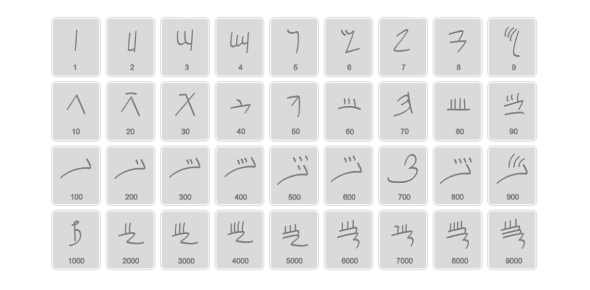# Ancient Numeral Systems Maths Quiz

10 Questions | Total Attempts: 143SettingsPlay this Maths quiz based on ancient numeral systems and check whether you know about the earliest number systems or not. The earliest numeral systems were used in the form of tally marks, initially written on stones and later on potters. Do you have any idea about such a system? If yes, take up the quiz below and test your knowledge. Also, it will make you aware of the history and basics of ancient number systems. Good luck, buddy!

• 1.
Which of the following is an ancient number system?
• A.

Cardinal

• B.

Babylonian

• C.

Binomial

• 2.
Which ancient number system uses 60 as the base?
• A.

Roman

• B.

Sumerian

• C.

None of the above

• 3.
The invention of numbers took place when people started .....
• A.

Writing books.

• B.

• C.

Eating.

• 4.
The clocks use ___ as a base for recording time.
• A.

60

• B.

100

• C.

20

• 5.
This is an example of _____ numeral system.
• A.

Sumerian

• B.

Chinese

• C.

Roman

• 6.
Egyptians used ______ pictures to denote numbers.
• A.

Hieroglyphic

• B.

Roman

• C.

Algebra

• 7.
This is an example of ______ number system.
• A.

Roman

• B.

Egyptian

• C.

Chinese

• 8.
Some historians think that 60 was used as a base because ______
• A.

It was easy to write the number 60.

• B.

It was divided by many numbers.

• C.

It was divided by 6.

• 9.
Each number in the Chinese number system was represented by a separate ____
• A.

Word

• B.

Symbol

• C.

Comma

• 10.
A _______ studies about life, events and objects related to ancient time.
• A.

Historian

• B.

Mathematician

• C.

GeologistBack to top